# Drawing graphics primitives¶

The pygame.draw module allows to draw simple shapes to a surface. This can be the screen surface or any Surface object such as an image or drawing:

• rectangle
• polygon
• circle
• ellipse

The functions have in common that they:

• take a Surface object as first argument
• take a color as second argument
• take a width parameter as last argument
• return a Rect object which bounds the changed area

the following format:

rect(Surface, color, Rect, width) -> Rect
polygon(Surface, color, pointlist, width) -> Rect
circle(Surface, color, center, radius, width) -> Rect


Most of the functions take a width argument. If the width is 0, the shape is filled.

## Draw solid and outlined rectangles¶

The following draws first the background color and then adds three overlapping solid rectangles and next to it three oulined overlapping rectangles with increasing line width:

screen.fill(background)
pygame.draw.rect(screen, RED, (50, 20, 120, 100))
pygame.draw.rect(screen, GREEN, (100, 60, 120, 100))
pygame.draw.rect(screen, BLUE, (150, 100, 120, 100))

pygame.draw.rect(screen, RED, (350, 20, 120, 100), 1)
pygame.draw.rect(screen, GREEN, (400, 60, 120, 100), 4)
pygame.draw.rect(screen, BLUE, (450, 100, 120, 100), 8)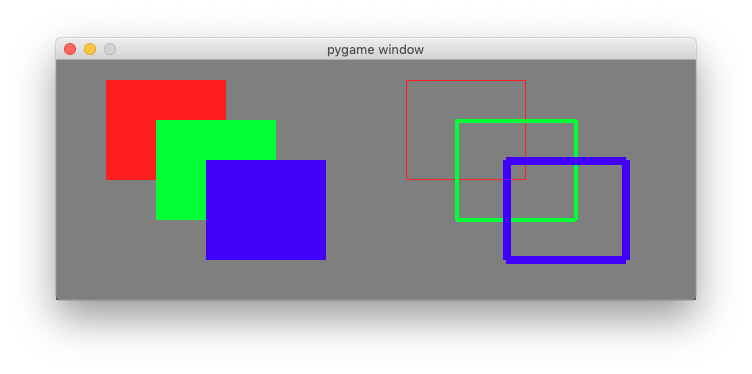Try to modifiy the parameters and play with the drawing function.

## Draw solid and outlined ellipses¶

The following code draws first the background color and then adds three overlapping solid ellipses and next to it three oulined overlapping ellipses with increasing line width:

screen.fill(background)
pygame.draw.ellipse(screen, RED, (50, 20, 160, 100))
pygame.draw.ellipse(screen, GREEN, (100, 60, 160, 100))
pygame.draw.ellipse(screen, BLUE, (150, 100, 160, 100))

pygame.draw.ellipse(screen, RED, (350, 20, 160, 100), 1)
pygame.draw.ellipse(screen, GREEN, (400, 60, 160, 100), 4)
pygame.draw.ellipse(screen, BLUE, (450, 100, 160, 100), 8)

pygame.display.update()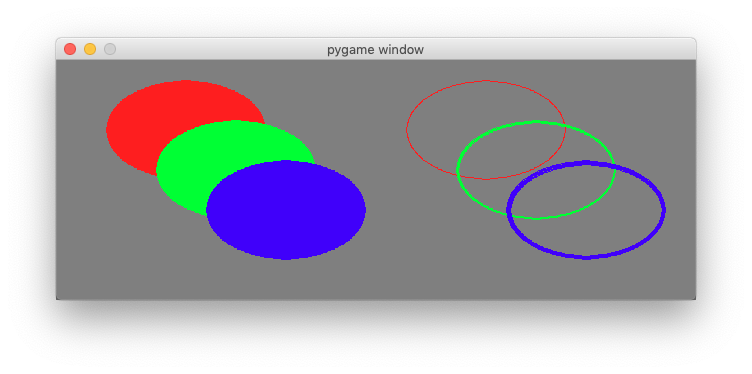draw2.py

## Detect the mouse¶

Pressing the mouse buttons produces MOUSEBUTTONDOWN and MOUSEBUTTONUP events. The flollowing code in the event loop detects them and writes the event to the console:

for event in pygame.event.get():
if event.type == QUIT:
running = False
elif event.type == MOUSEBUTTONDOWN:
print(event)
elif event.type == MOUSEBUTTONUP:
print(event)


Pressing the mouse buttons produces this kind of events:

<Event(5-MouseButtonDown {'pos': (123, 88), 'button': 1, 'window': None})>
<Event(6-MouseButtonUp {'pos': (402, 128), 'button': 1, 'window': None})>
<Event(5-MouseButtonDown {'pos': (402, 128), 'button': 3, 'window': None})>
<Event(6-MouseButtonUp {'pos': (189, 62), 'button': 3, 'window': None})>


Just moving the mouse produces a MOUSEMOTION event. The following code detects them an writes the event to the console:

elif event.type == MOUSEMOTION:
print(event)


Moving the mosue produces this kind of event:

<Event(4-MouseMotion {'pos': (537, 195), 'rel': (-1, 0), 'buttons': (0, 0, 0), 'window': None})>
<Event(4-MouseMotion {'pos': (527, 189), 'rel': (-10, -6), 'buttons': (0, 0, 0), 'window': None})>
<Event(4-MouseMotion {'pos': (508, 180), 'rel': (-19, -9), 'buttons': (0, 0, 0), 'window': None})>


## Draw a rectangle with the mouse¶

We can use this three events to draw a rectangle on the screen. We define the rectangle by its diagonal start and end point. We also need a flag which indicates if the mouse button is down and if we are drawing:

start = (0, 0)
size = (0, 0)
drawing = False


When the mouse button is pressed, we set start and end to the current mouse position and indciate with the flag that the drawing mode has started:

elif event.type == MOUSEBUTTONDOWN:
start = event.pos
size = 0, 0
drawing = True


When the mouse button is released, we set the end point and indicate with the flag that the drawing mode has ended:

elif event.type == MOUSEBUTTONUP:
end = event.pos
size = end - start, end - start
drawing = False


When the mouse is moving we have also have to check if we are in drawing mode. If yes, we set the end position to the current mouse position:

elif event.type == MOUSEMOTION and drawing:
end = event.pos
size = end - start, end - start


Finally we draw the rectangle to the screen. First we fill in the background color. Then we calculate the size of the rectangle. Finally we draw it, and at the very last we update the screen:

screen.fill(GRAY)
pygame.draw.rect(screen, RED, (start, size), 2)
pygame.display.update()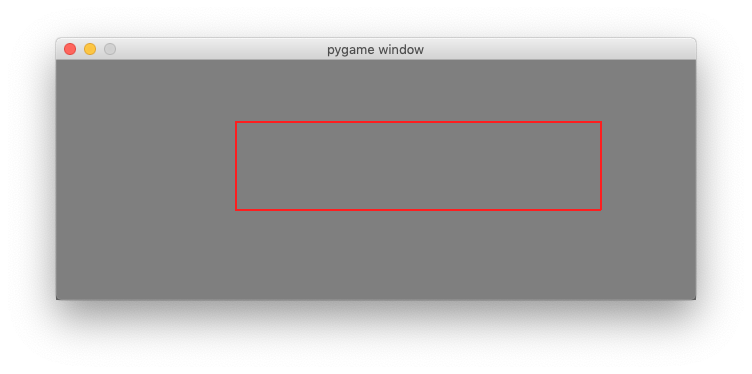mouse2.py

## Draw multiple shapes¶

To draw multiple shapes, we need to place them into a list. Besides variables for start, end and drawing we add a rectangle list:

start = (0, 0)
size = (0, 0)
drawing = False
rect_list = []


When drawing of an object (rectangle, circle, etc.) is done, as indicated by a MOUSEBUTTONUP event, we create a rectangle and append it to the rectangle list:

elif event.type == MOUSEBUTTONUP:
end = event.pos
size = end-start, end-start
rect = pygame.Rect(start, size)
rect_list.append(rect)
drawing = False


In the drawing code, we first fill the background color, then iterate through the rectagle list to draw the objects (red, thickness=3), and finally we draw the current rectangle which is in the process of being drawn (blue, thickness=1):

screen.fill(GRAY)
for rect in rect_list:
pygame.draw.rect(screen, RED, rect, 3)
pygame.draw.rect(screen, BLUE, (start, size), 1)
pygame.display.update()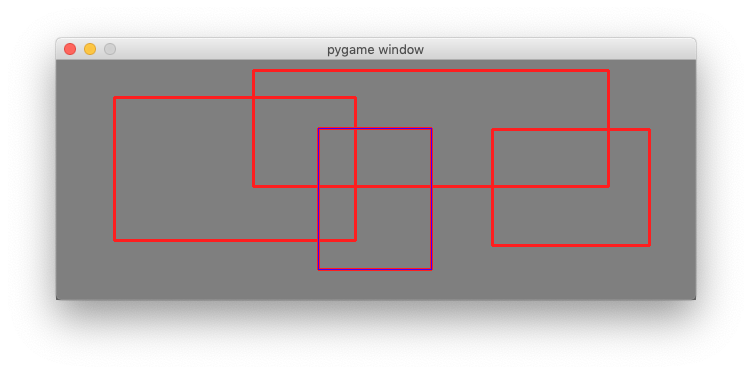Here is the complete file:

"""Place multiple rectangles with the mouse."""

import pygame
from pygame.locals import *

RED = (255, 0, 0)
BLUE = (0, 0, 255)
GRAY = (127, 127, 127)

pygame.init()
screen = pygame.display.set_mode((640, 240))

start = (0, 0)
size = (0, 0)
drawing = False
rect_list = []

running = True

while running:
for event in pygame.event.get():
if event.type == QUIT:
running = False

elif event.type == MOUSEBUTTONDOWN:
start = event.pos
size = 0, 0
drawing = True

elif event.type == MOUSEBUTTONUP:
end = event.pos
size = end-start, end-start
rect = pygame.Rect(start, size)
rect_list.append(rect)
drawing = False

elif event.type == MOUSEMOTION and drawing:
end = event.pos
size = end-start, end-start

screen.fill(GRAY)
for rect in rect_list:
pygame.draw.rect(screen, RED, rect, 3)
pygame.draw.rect(screen, BLUE, (start, size), 1)
pygame.display.update()

pygame.quit()


mouse3.py

## Draw a pologyon line with the mouse¶

To draw a polygon line we need to add the points to a list of points. First we define an empty point list and a drawing flag:

drawing = False
points = []


At the MOUSEBUTTONDOWN event we add the current point to the list and set the drawing flag to True:

elif event.type == MOUSEBUTTONDOWN:
points.append(event.pos)
drawing = True


At the MOUSEBUTTONUP event we deactivate the drawing flag:

elif event.type == MOUSEBUTTONUP:
drawing = False


At the MOUSEMOTION event we move the last point in the polygon list if the drawing flag is set:

elif event.type == MOUSEMOTION and drawing:
points[-1] = event.pos


If there are more than 2 points in the point list we draw a polygon line. Each pygame.draw function returns a Rect of the bounding rectangle. We display this bounding rectangle in green:

screen.fill(GRAY)
if len(points)>1:
rect = pygame.draw.lines(screen, RED, True, points, 3)
pygame.draw.rect(screen, GREEN, rect, 1)
pygame.display.update()


Pressing the ESCAPE key will remove the last point in the list:

elif event.type == KEYDOWN:
if event.key == K_ESCAPE:
if len(points) > 0:
points.pop()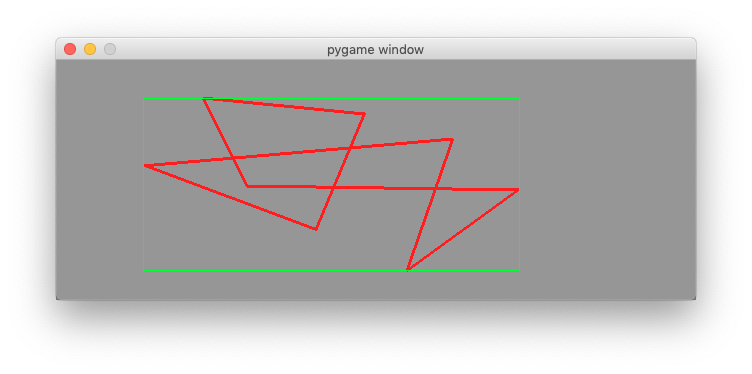Here is the complete file:

"""Place a polygone line with the clicks of the mouse."""

import pygame
from pygame.locals import *

RED = (255, 0, 0)
GREEN = (0, 255, 0)
GRAY = (150, 150, 150)

pygame.init()
screen = pygame.display.set_mode((640, 240))

drawing = False
points = []
running = True

while running:
for event in pygame.event.get():
if event.type == QUIT:
running = False

elif event.type == KEYDOWN:
if event.key == K_ESCAPE:
if len(points) > 0:
points.pop()

elif event.type == MOUSEBUTTONDOWN:
points.append(event.pos)
drawing = True

elif event.type == MOUSEBUTTONUP:
drawing = False

elif event.type == MOUSEMOTION and drawing:
points[-1] = event.pos

screen.fill(GRAY)
if len(points)>1:
rect = pygame.draw.lines(screen, RED, True, points, 3)
pygame.draw.rect(screen, GREEN, rect, 1)
pygame.display.update()

pygame.quit()


mouse4.py